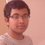# Cartesian Product!

We know that given two non - void sets, say $A$ and $B$, their $\textbf{Cartesian Product}$ is defined as $A\,\times\,B\,=\,\{(a,b)\,:\,a\,\in\,A\,,\,b\,\in\,B\}$. For eg. If $A\,=\,\{1,3\}$ and $B\,=\,\{2,4,9\}$, then their Cartesian product is defined as $\{(1,2),(1,4),(1,9),(3,2),(3,4),(3,9)\}$.

I was wondering what if the set $A$ itself consists of ordered pairs, like what if $A\,=\,\{(1,5),(1,9),(2,5)\}$ and say $B\,=\,\{3,7\}$, then how do we define the $A\,\times B$?

One thing that I've observed is that ,in case, if the set that consists of ordered pairs ( n-tuples in general) can be expressed as Cartesian product of simple sets (sets that consists of numbers only), then the overall cartesian product can be found. What I mean is

Say, $A\,=\,\{(3,4),(3,7),(2,4),(2,7)\}$ and $B\,=\,\{2,5\}$ and $A\times B$ is to be found, then one can proceed as follows :

$A\times B\,=\,\{(3,4),(3,7),(2,4),(2,7)\}\,\times\,\{2,5\}\,\,=\,\{3,2\}\,\times\,\{4,7\}\,\times\,\{2,5\}\,\,=\,\{(2,3,4),(2,3,7),(2,2,4),(2,2,7),(5,3,4),(5,3,7),(5,2,4),(5,2,7)\}$.Note by Aditya Sky
4 years, 3 months ago

This discussion board is a place to discuss our Daily Challenges and the math and science related to those challenges. Explanations are more than just a solution — they should explain the steps and thinking strategies that you used to obtain the solution. Comments should further the discussion of math and science.

When posting on Brilliant:

• Use the emojis to react to an explanation, whether you're congratulating a job well done , or just really confused .
• Ask specific questions about the challenge or the steps in somebody's explanation. Well-posed questions can add a lot to the discussion, but posting "I don't understand!" doesn't help anyone.
• Try to contribute something new to the discussion, whether it is an extension, generalization or other idea related to the challenge.
• Stay on topic — we're all here to learn more about math and science, not to hear about your favorite get-rich-quick scheme or current world events.

MarkdownAppears as
*italics* or _italics_ italics
**bold** or __bold__ bold
- bulleted- list
• bulleted
• list
1. numbered2. list
1. numbered
2. list
Note: you must add a full line of space before and after lists for them to show up correctly
paragraph 1paragraph 2

paragraph 1

paragraph 2

[example link](https://brilliant.org)example link
> This is a quote
This is a quote
    # I indented these lines
# 4 spaces, and now they show
# up as a code block.

print "hello world"
# I indented these lines
# 4 spaces, and now they show
# up as a code block.

print "hello world"
MathAppears as
Remember to wrap math in $$ ... $$ or $ ... $ to ensure proper formatting.
2 \times 3 $2 \times 3$
2^{34} $2^{34}$
a_{i-1} $a_{i-1}$
\frac{2}{3} $\frac{2}{3}$
\sqrt{2} $\sqrt{2}$
\sum_{i=1}^3 $\sum_{i=1}^3$
\sin \theta $\sin \theta$
\boxed{123} $\boxed{123}$

## Comments

Sort by:

Top Newest

Do the same thing. $A \times B = \{ ( a, b) | a \in A, b \in B \}$. It doesn't matter what the sets $A$ and $B$ are.

E.g. you can determine $\{ \text{ elephant, zinc} \} \times \{ \frac{1}{2} , ( 1, 2) \}$.

Staff - 4 years, 3 months ago

Log in to reply

OK...

- 4 years, 3 months ago

Log in to reply

Infact, this is the method followed to generate n-ary relations.......

- 2 years, 3 months ago

Log in to reply

×

Problem Loading...

Note Loading...

Set Loading...